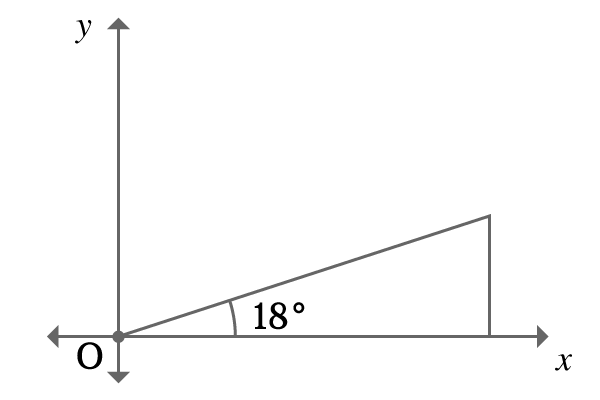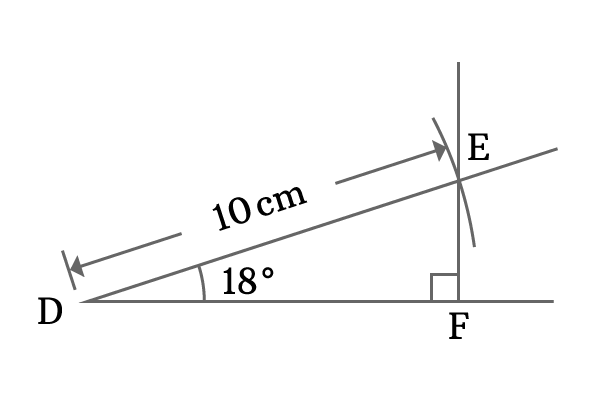# $\sin{(18^°)}$ Proof

The value of sin function for an angle 18 is called $\sin{18^\circ}$.

$\sin{18^\circ} = \dfrac{\sqrt{5} -1}{4} = 0.309016994\ldots$

### Trigonometric method

The value of sine $18$ degrees can be derived in fraction in trigonometry by using trigonometric formulae.

#### Technique to split angle as compound angle

Take $\theta = 18^\circ$. Therefore $5 \theta = 90^\circ$.

Divide the angle $5\theta$ as sum of two angles and it is better to write the compound angle as the sum of $2\theta$ and $3\theta$.

$2\theta + 3\theta = 90^\circ$

$\implies$ $2\theta = 90^\circ -3\theta$

Take sine both sides to express compound angle in terms of trigonometric functions.

$\implies$ $\sin{2\theta} = \sin{(90^\circ-3\theta)}$

$\implies$ $\sin{2\theta} = \cos{3\theta}$

#### Use expansion of trigonometric functions

$\sin{2\theta}$ and $\cos{3\theta}$ can be expanded by double and triple angle identities.

$\implies$ $2\sin{\theta}\cos{\theta}$ $=$ $4\cos^3{\theta} -3\cos{\theta}$

$\implies$ $0$ $=$ $4\cos^3{\theta} -3\cos{\theta}$ $-2\sin{\theta}\cos{\theta}$

$\implies$ $4\cos^3{\theta} -3\cos{\theta}$ $-2\sin{\theta}\cos{\theta} = 0$

$\cos{\theta}$ is a common multiplying factor of each term in this equation. So, take it common from them to simplify the equation further.

$\implies$ $\cos{\theta}{(4\cos^2{\theta}-3-2\sin{\theta})}$ $=$ $0$

#### Further simplification of the trigonometric equation

Express cosine term in terms of sine to make second multiplying factor as a quadratic equation. It can be done by converting square of cosine function as a square of sine function as per Pythagorean identity of sine and cosine functions.

$\implies$ $\cos{\theta} (4(1 -\sin^2{\theta}) -3-2\sin{\theta})$ $= 0$

$\implies$ $\cos{\theta} (4 -4\sin^2{\theta} -3-2\sin{\theta})$ $= 0$

$\implies$ $\cos{\theta} (-4\sin^2{\theta} -2\sin{\theta} + 1)$ $= 0$

$\implies$ $\cos{\theta} (4\sin^2 \theta + 2\sin \theta -1)$ $= 0$

#### Solving the trigonometric equation

Solve the trigonometric equation to obtain the value of sine $18$ degrees in trigonometrical approach.

$\cos{\theta} = 0$ and $4\sin^2{\theta} + 2\sin{\theta} -1 = 0$

The value of angle $\theta$ is taken as $18^\circ$. So, cosine of angle $18^\circ$ should be equal to zero but $\cos{90^\circ} = 0$. So, it is not possible. Therefore, $\cos{18^\circ} \ne 0$. Therefore, ignore $\cos{\theta} = 0$ equation.

Now consider the second multiplying factor and it is $4\sin^2 \theta + 2\sin \theta -1 = 0$. It is a quadratic equation and solve it by quadratic formula method.

$\sin{\theta} = \dfrac{-2 \pm \sqrt{(2)^2 -4 \times 4 \times (-1)}}{2 \times 4}$

$\implies$ $\sin{\theta} = \dfrac{-2 \pm \sqrt{4 + 16}}{8}$

$\implies$ $\sin{\theta} = \dfrac{-2 \pm \sqrt{20}}{8}$

$\implies$ $\sin{\theta} = \dfrac{-2 \pm 2\sqrt{5}}{8}$

$\implies$ $\sin{\theta} = \dfrac{-1 \pm \sqrt{5}}{4}$

$\,\,\, \therefore \,\,\,\,\,\,$ $\sin{\theta} = \dfrac{-1 -\sqrt{5}}{4}$ and $\sin{\theta} = \dfrac{-1 + \sqrt{5}}{4}$

#### Determining true value of sine 18 degrees

$\sin{\theta}$ $=$ $\dfrac{-1-\sqrt{5}}{4} = -0.809016994\ldots$

$\sin{\theta}$ $=$ $\dfrac{\sqrt{5}-1}{4} = 0.309016994\ldots$

The trigonometric equation has given two solutions. One is negative and second one is positive. One of them is true possibly and it is not possible that sine function gives two values for angle $18^\circ$.Logically, it can be determined. Draw a right angled triangle with $18$ degrees and the triangle should be in first quadrant.

The lengths of opposite side and hypotenuse are positive in first quadrant. So, the ratio of lengths of opposite side to hypotenuse should also be positive and the ratio is known as sine function for that angle.

$\sin{18^\circ}$ $\ne$ $\dfrac{-1-\sqrt{5}}{4}$

$\,\,\, \therefore \,\,\,\,\,\,$ $\sin{18^\circ}$ $=$ $\dfrac{\sqrt{5}-1}{4}$

It is a value of sin function for angle $18^\circ$ in fraction.

### Geometrical Method

The value of sine $18$ degrees can also be determined in geometric method but it gives an approximate value and it is useful to us to verify the exact value of sine $18$ degrees, obtained from trigonometric approach.1. Draw a horizontal line from point $D$ by ruler.
2. Draw $18$ degrees line from point $D$ by using protractor and ruler.
3. Set compass to any length with ruler. It is set to $10 \, cm$ in this example. Draw an arc on $18$ degrees line from point $D$ and intersects the $18^\circ$ line at point $E$.
4. Finally, draw a perpendicular line to horizontal line from point $E$ and it intersects the horizontal line at point $F$.

Thus, a right angled triangle ($\Delta FDE$) with angle $18^\circ$ is formed geometrically. Now, calculate the value of sin 18 by calculating the ratio of lengths of opposite side to hypotenuse.

In this case, the length of hypotenuse is $10 \, cm$ but the length of opposite side is unknown. However, it can be measured by ruler. You observe that it will be $3.1 \, cm$. Now, calculate the value of $\sin{18^\circ}$.

$\sin 18^\circ = \dfrac{FE}{DE}$

$\implies \sin{18^\circ} = \dfrac{3.1}{10} = 0.31$

#### Conclusion

The value of $\sin{18^\circ}$ is $0.309016994\ldots$ in trigonometric method but the value of $\sin{18^\circ}$ is $0.31$ geometrically. The values of both methods are almost same but value of $\sin{18^\circ}$ derived from trigonometric method is exact value and it is an irrational number. The value of $\sin{18^\circ}$ derived from geometric method is an approximate value and it is not possible to obtain the exact value of sin $18$ degrees in direct geometric method.

A best free mathematics education website that helps students, teachers and researchers.

###### Maths Topics

Learn each topic of the mathematics easily with understandable proofs and visual animation graphics.

###### Maths Problems

A math help place with list of solved problems with answers and worksheets on every concept for your practice.

Learn solutions

###### Subscribe us

You can get the latest updates from us by following to our official page of Math Doubts in one of your favourite social media sites.